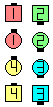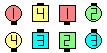Definitions of Square Dance Calls and Concepts

Index -->  Plus  |  A1  |  A2  |  C1  |  C2  |  C3A  |  C3B  |  C4  |  NOL  |
Definitions (Text Only) -->  Plus  |  A1  |  A2  |  C1  |  C2  |  C3A  |  C3B  |  C4  |  NOL  |
 Find call:

\$B30B&\$,(B Column \$B\$N(B 2 x 4 \$B\$^\$?\$OB>\$NE,@Z\$J(B formation \$B\$+\$i!%(B

Centers \$B\$O(B Phantom Run (Reverse Flip) \$B\$r(B, \$B30B&\$N?M\$O(B Column Circulate twice \$B\$r\$7\$^\$9!%(B

Columns \$B\$+\$i\$OJ?9T\$J(B Line \$B\$K\$J\$j\$^\$9!%(BExpand The Column\$B\$NA0(B \$B8e(B

Magic Expand The Column [C3A]: Centers \$B\$O(B Phantom Run (Reverse Flip) \$B\$r(B, \$B30B&\$N?M\$O(B Magic Column Circulate twice \$B\$r\$7\$^\$9!%(B

Cross Expand The Column [NOL]: Centers \$B\$O(B Trade & Spread (Reverse Cut) \$B\$r(B, \$B30B&\$N?M\$O(B Column Circulate twice \$B\$r\$7\$^\$9!%(B

Expand The Butterfly [NOL]: Butterfly \$B\$+\$i!%(B Centers \$B\$O(B Phantom Run \$B\$r(B, Ends \$B\$O(B Butterfly Circulate twice \$B\$r\$7\$^\$9!%(B

\$BDisband [C3B].CALLERLAB definition for Expand The ColumnChoreography for Expand The ColumnComments? Questions? Suggestions?

https://www.ceder.net/def/expandthecolumn.php?level=master&language=japan
25-November-2020 16:59:39